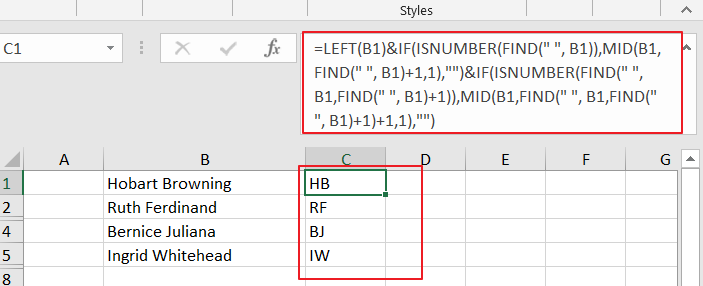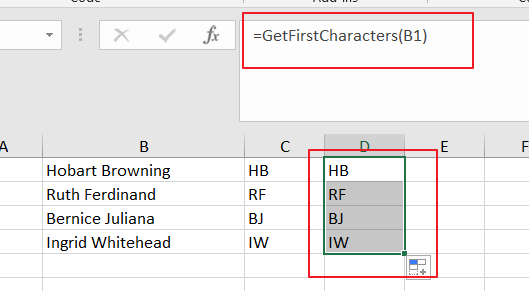# How to Extract Initials From a Name in Excel

This post will guide you how to get initials from a given name using a formula in Excel. How do I extract initials from names in Excel 2013/2016.

Assuming that you have a list of data in range B1:B4, in which contain name values, and you want to extract initials from these names. How to accomplish it. this post will show you two methods to get initials.

## Extract Initials from a Name Using a Formula

To extract initials from a given name in Excel, you can do this using a formula based on the LEFT function, the IF function, the ISNUMBER function and the MID function. Like this:

=LEFT(B1)&IF(ISNUMBER(FIND(” “, B1)),MID(B1,FIND(” “, B1)+1,1),””)&IF(ISNUMBER(FIND(” “, B1,FIND(” “, B1)+1)),MID(B1,FIND(” “, B1,FIND(” “, B1)+1)+1,1),””)

You need to type this formula into a blank cell and press Enter key on your keyboard, and then drag the AutoFill Handle down to other cells.## Extract Initials from a Name Using User Defined Function

You can also define a User Defined function to extract initials from a given name in Excel. Just do the following steps:

Step1# open your excel workbook and then click on “Visual Basic” command under DEVELOPER Tab, or just press “ALT+F11” shortcut.Step2#  then the “Visual Basic Editor” window will appear.

Step3# click “Insert” ->”Module” to create a new module.Step4# paste the below VBA code into the code window. Then clicking “Save” button.

```Function GetFirstCharacters(pWorkRng As Range) As String
myValue = pWorkRng.Value
myStr = VBA.Split(Trim(myValue))
For i = 0 To UBound(myStr)
myResult = myResult & VBA.Left(myStr(i), 1) & ""
Next
GetFirstCharacters = myResult
End Function
```

Step5# type the following formula into a blank cell and press Enter key in your keyboard.

=GetFirstCharacters(B1)### Related Functions

• Excel IF function
The Excel IF function perform a logical test to return one value if the condition is TRUE and return another value if the condition is FALSE. The IF function is a build-in function in Microsoft Excel and it is categorized as a Logical Function.The syntax of the IF function is as below:= IF (condition, [true_value], [false_value])….
• Excel LEFT function
The Excel LEFT function returns a substring (a specified number of the characters) from a text string, starting from the leftmost character.The LEFT function is a build-in function in Microsoft Excel and it is categorized as a Text Function.The syntax of the LEFT function is as below:= LEFT(text,[num_chars])…t)…
• Excel ISNUMBER function
The Excel ISNUMBER function returns TRUE if the value in a cell is a numeric value, otherwise it will return FALSE.The syntax of the ISNUMBER function is as below:= ISNUMBER (value)…
• Excel MID function
The Excel MID function returns a substring from a text string at the position that you specify.The syntax of the MID function is as below:= MID (text, start_num, num_chars)…
Related Posts

Abbreviate Names Or Words in Excel

As an MS Excel user, you might have come across a task where you need to abbreviate different names or words, and there are also possibilities that you might have done this task manually by assuming that there isn't any ...

Extract Last Two Words From Multiple Cells

Just assume that you have a few cells containing values/words and you want to extract the last two words from each cell into another separate cell; then you might think that it's not a big deal; because you would prefer ...

Extract Multiple Lines From A Cell

Suppose that you have listed some text in a single cell which is separated by the line break(you can do it by pressing ALT + ENTER after entering the text), and now you want to extract multiple lines of text ...

Extract substring In Excel

This post will guide you how to use Excel's MID function is a quick and easy way to extract pieces from your text. Use the Excel formula to extract a substring with MID. Note: If you want to extract just ...

How to Count Numbers Nth Digit Equals to Specific Number in Excel

This post will guide you how to count numbers where the Nth digit equal to a specific number in Excel 2013/2016 or Excel office 365. How do I count of numbers in a given range where the Nth digit is ...

How to Convert Text to Time in Excel

This post will guide you how to convert time string to time in Excel. Assuming that you have a list of text string in your worksheet, and you wish to convert these time string to standard time format, how to ...

How to Extract Number from Text String in Excel

This post will guide you how to extract number from a given test string in Excel. How do I extract all numbers from string using a formula in Excel. How to get all number from a given test string using ...

How to Convert mmddyy to Date in Excel

This post will guide you how to convert non-standard date formats or text to a standard date in Excel. If you have a date with mmddyy format, and you want to change it as the normal date format as mm/dd/yyyy ...

How to Convert Military Time to Standard Time in Excel

This post will guide you how to convert military time to standard time with a formula in Excel. How do I convert from military time 17:29:30 to regular time 5:29:30PM in Excel.  How do I Convert Standard time to military ...

How to Extract Text between Two Text Strings in Excel

This post will guide you how to extract text between two given text strings in Excel. How do I get text string between two words with a formula in Excel. Extract Text between Two Text Strings Assuming that you have ...

Sidebar